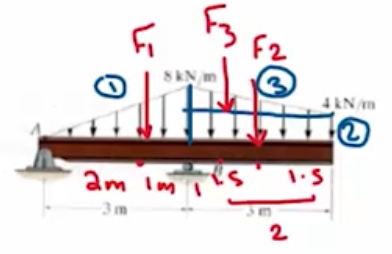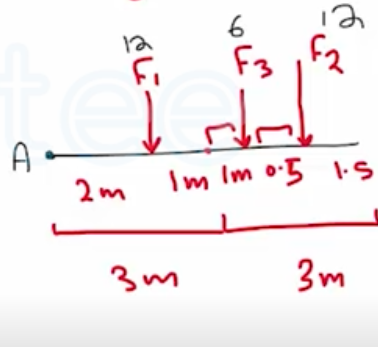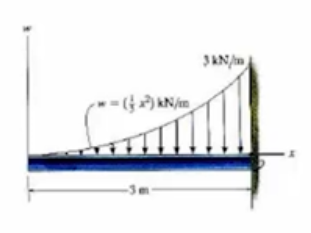Need Help?

Subscribe to Statics

###### \${selected_topic_name}
• Notes

Replace the distributed loading with an equivalent  resultant force, and specify its location on the beam  measured from point  $A$.

$F_{1}=\left(\frac{1}{2} * 3 * 8\right)=12 k N$$\frac{3}{3}=1$

$F_{2}=(3 * 4)=12 k N$

$F_{3}=\left(\frac{1}{2} * 3*4\right)=6 \mathrm{kN}$

$F_{R}=12+12+6=30 \mathrm{kN} \downarrow$$\sum M_{A}=(12 * 2)+(6 * 4)+(12*4 \cdot 5)$

$=102 kN.m$

$=F_R*d$

$d=3.4m$

Determine the equivalent resultant force and couple moment at point  O .$F=\int_{0}^{3} w d x$

$=\int_{0}^{3} \frac{1}{3} x^{2} d x=\left[\frac{x^{3}}{9}\right]_{0}^{5}=3 k N \downarrow$

$M=\int_{0}^{3}(w \widetilde{x}) d x$

$=\int_{0}^{3}\left(\frac{1}{3} x^{2}\right)(3-x) d x$

$=\int_{0}^{3}\left(x^{2}-\frac{x^{3}}{3}\right) d x =\left[\frac{x^{3}}{3}-\frac{x^{4}}{12}\right]_{0}^{3}=2.25 \mathrm{kNm}$